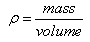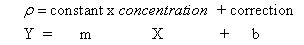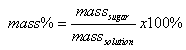How much sugar is in the soda?

You are a technician in the well known 'Soda Beverage Industry Quality Control Center' (SBIQCC) and are tasked with testing and reporting mass% of sugar in two different samples (the one you bring in and the one your partner brings into lab) to make sure that the consumer is getting as much teeth-destroying sugar they paid for.

The purpose of the experiment (the first part of your lab report) is to experimentally determine the mass% of sugar in two soda samples and compare it to the nutritional facts labs on said sodas.

Density is the mass of a substance per unit volume. Density is typically expressed in grams per milliliter, which is equal to grams per cubic centimeter. Because density is an intensive property, it is useful for identifying the composition of a substance.

As any coffee drinker knows, sugar dissolves readily in water. The density of a solution depends on the amount of sugar dissolved in the solution. In this experiment, you will determine the density of some solutions of known concentration, and obtain a calibration curve, which will allow you to determine the percent of sugar in an your soda sample. Density is easily calculated using the equationAs we add sugar to a solution, the density of the solution should go up in a proportional (linear) fashion. Put mathematically,If we plot a graph of mass % versus density, we should get a straight line graph with a very useful equation. That equation will give us the numerical relation between density and concentration. We can then use that equation to determine the mass % of sugar in the soda solution by simply measuring the density and calculating mass %, using this equation.

By definition,; Where masssolution = masssugar + masswater

You will measure out a particular volume of liquid and obtain its mass (of solution), allowing you to solve for density. Everyone in lab will be making a few solutions with various amount of sugar (from 0% to 15% by mass) All of the data will be collected and everyone will make a Graph of the data (go here for instructions if using Office 07) of mass % vs. density, including the equation and R2 values .

To make the known solutions of  sugar, you will need to know the mass of sugar and the mass of water placed in a container of known volume. The container you will be using is a volumetric flask (if you do not know what one is, follow that link!) Into a clean flask, you will place a known mass of sugar, and then add water and stir until it is all dissolved. The dissolved solution is then diluted ‘to the mark’. You measure the mass of the flask filled with water and you can then determine the mass of water added (to get the mass of water). With masssugar and masswater you can determine the mass % of sugar in solution. Since you know the volume you diluted the sample to you can then determine density of the solution (make sure you use masssolution in your calculation.)

You will then measure the density of your unknown sample (see prelab questions) plugging the measured value of density into you correlation equation (in the graph) will give the mass % of the unknown solution.You can then compare this number to the can of soda. In the nutritional facts label there is an listing of the g of sugar in the whole soda. Knowing the density of the soda, you can determine the mass of soda in the can (because you know the volume) and thus the mass % that the company says should be in the soda.

Your lab report will be of short form memo style to your supervisor at SBIQCC. Of course, it will include your correlation chart (there should be no 'y' or 'x in your calculation. This is not math class, you have a mass% axis and a density axis, not x and y) complete with equation (no x nor y!), Sample mass% and density calculations of the known solutions as well as your determination of yours and your partners soda samples should be in the attachment . Make sure you include everything else that is needed. The procedure, if written correctly could be only 2-3 sentences long. In your conclusion section you will discuss the difference between your value and what the nutritional facts label says.

Pre lab questions: (to be completed on a separate piece of labeled recycled paper)

1. Bring to class a room temperature (cold = bad!) container of soda or juice that contains some amount of sugar (no diet soda) This must be a commercial solution, not a batch of sugar water you made up at home. You will NOT be consuming these in lab. The container MUST have a nutrional facts label.

2. In a run of the experiment, I measure the mass of an empty 50 mL flask (33.044 g) After I put in some sugar, the mass of flask + sugar is 35.366 g. I then add about 35 mL of water to the flask and gently shake until it all dissolves. I then dilute it to the mark (such that the total solution volume is exactly 50.00 mL) and measure the mass again. The mass of flask + sugar + water = 85.136 g. Calculate the mass % and density of this known solution. (hints: how do you calculate the mass of sugar in the solution?)
3. Assuming the denisty of your soda sample (prelab #1) is 1.033 g/mL, use the nutritional facts label to calculate the mass % of sugar in your soda sample.

On a different piece of recycled paper, come up with an appropriate data sheet for this experiment (what do you need to measure and write down?) Show it to your instructor as you enter lab.

Last Updated by MEO :29Dec10